# Variable g Pendulum

\$150.00

Code Number: P041213

Brand: AELAB

#### Purpose:

Measure the period of an oscillating pendulum as a function of the effective component of the gravitational acceleration

- +

Variable g pendulum is used to investigate the oscillation behaviour of a pendulum (rod pendulum) by varying the magnitude of the components of the acceleration of gravity which are decisive for the oscillation period. The pendulum that is to be used is constructed in such a manner that its oscillation plane can be progressively rotated from a vertical orientation to a horizontal one.

Pendulum with continuously adjustable plane of oscillation for observing pendulum oscillations where the acceleration due to gravity g can appear to be varied.

Max. length of pendulum:450 mm
Mass of pendulum: 0.2 kg
Angle of plane of oscillation: 0° – 90°

1. Measurement of the oscillation period of the variable g pendulum as a function of the angle of inclination α of the oscillation plane for two different pendulum lengths.

2. Graphical analysis of the measured correlations and a comparison with the theoretical curves, which have been standardised with the measured value at α = 0.

3. Calculation of the effective pendulum length L for the acceleration of gravity, which is assumed to be known. Comparison of this value with the distance between the pivot point of the pendulum and the centre of gravity of the mobile pendulum weight.

4. On the moon’s surface the “lunar acceleration of gravity” gm is only 16.6% of the earth’s acceleration of gravity g. Calculate the angle α and set it on the device such that the pendulum in the laboratory oscillates with the same oscillation period with which it would oscillate on the moon in a perpendicular position. Compare the measured oscillation period with the calculated one.

This set includes:

1. Variable g pendulum

2. Stainless steel rod 8mm, length 45cm, threaded end.

3. Support rod 16mm, length 60cm.

4. Weight 110g

5. H-Shaped Base (option)

6. Digital Timer with light barrier (Option)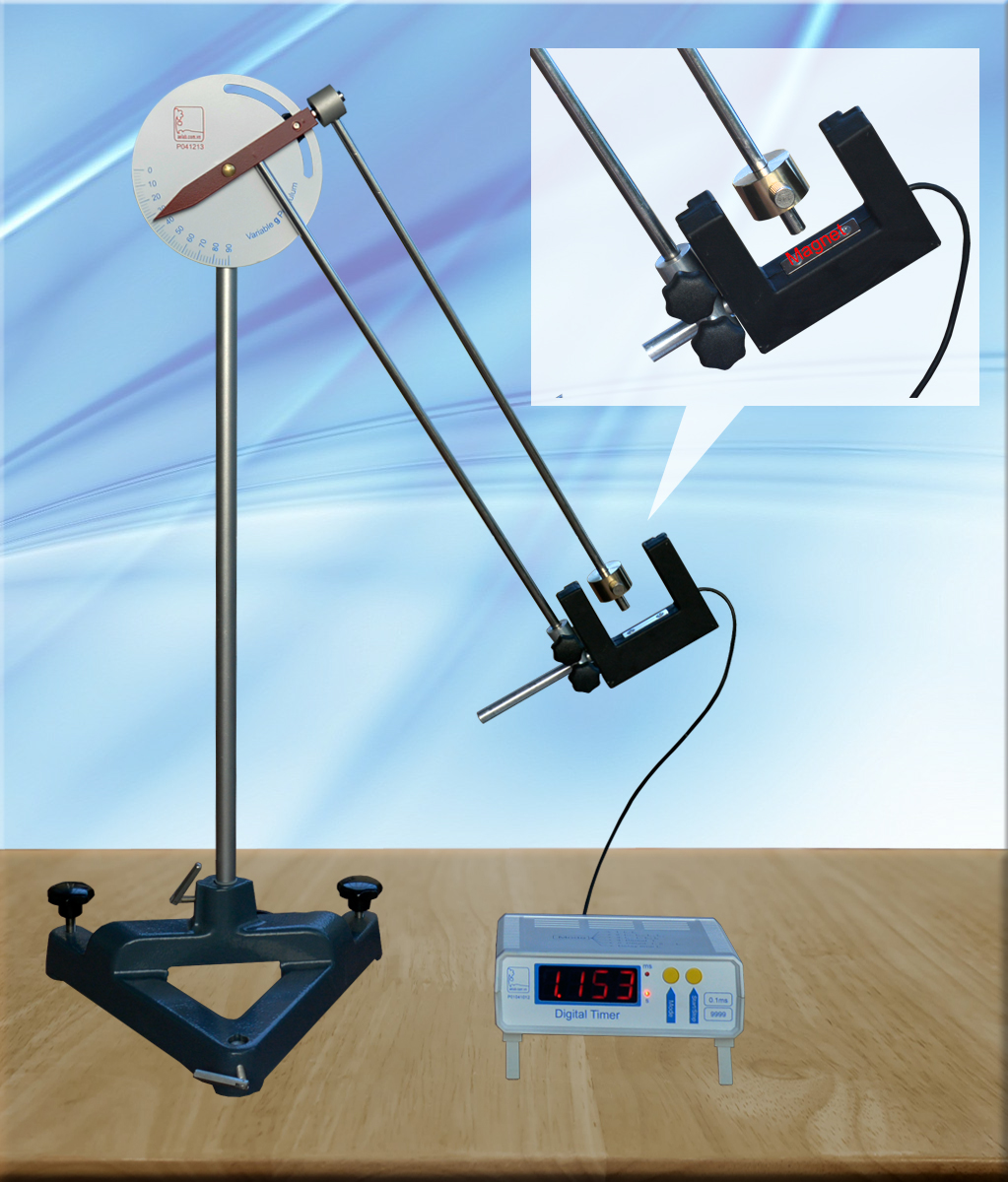Variable g pendulum with timer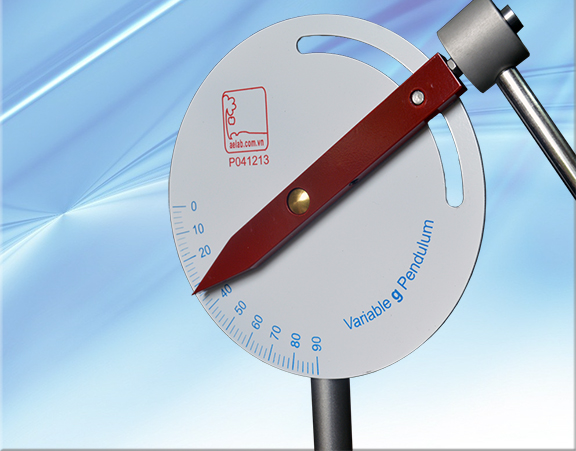variable g pendulum: Angle scale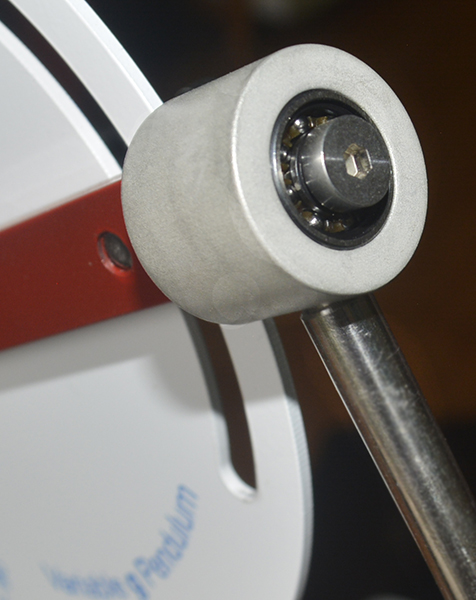variable g pendulum: Ball bear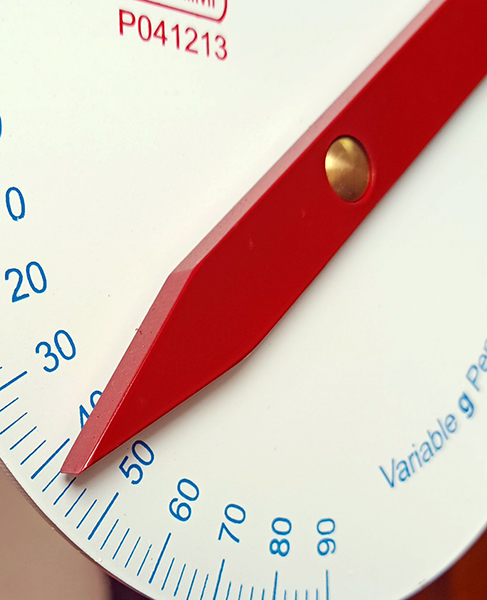Variable g pendulum: Needle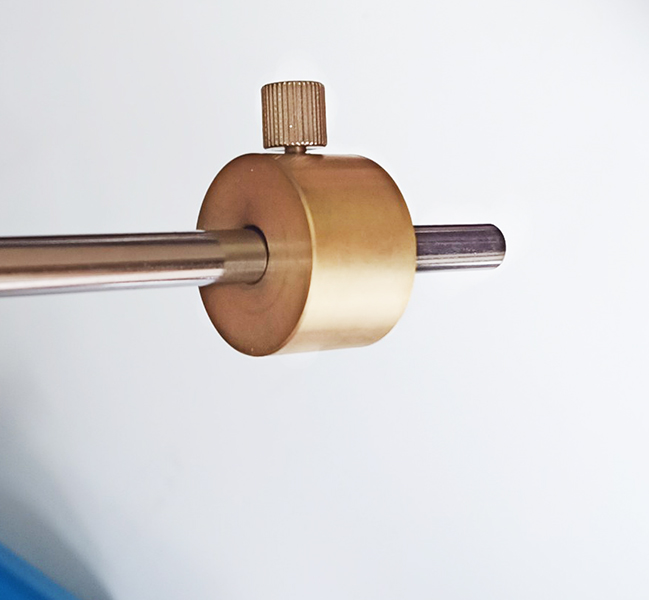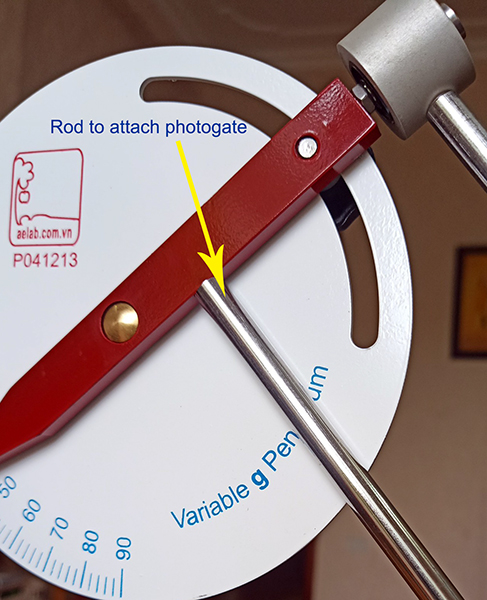When the axis is tilted, the component of the gravitational acceleration g that is parallel to the axis gpar is rendered ineffective by the fact that the axis is fixed (see fig. below). The
remaining component that is effective geff is given by the following equation: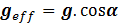(1)

The period of oscillation of the pendulum: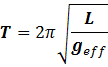(2)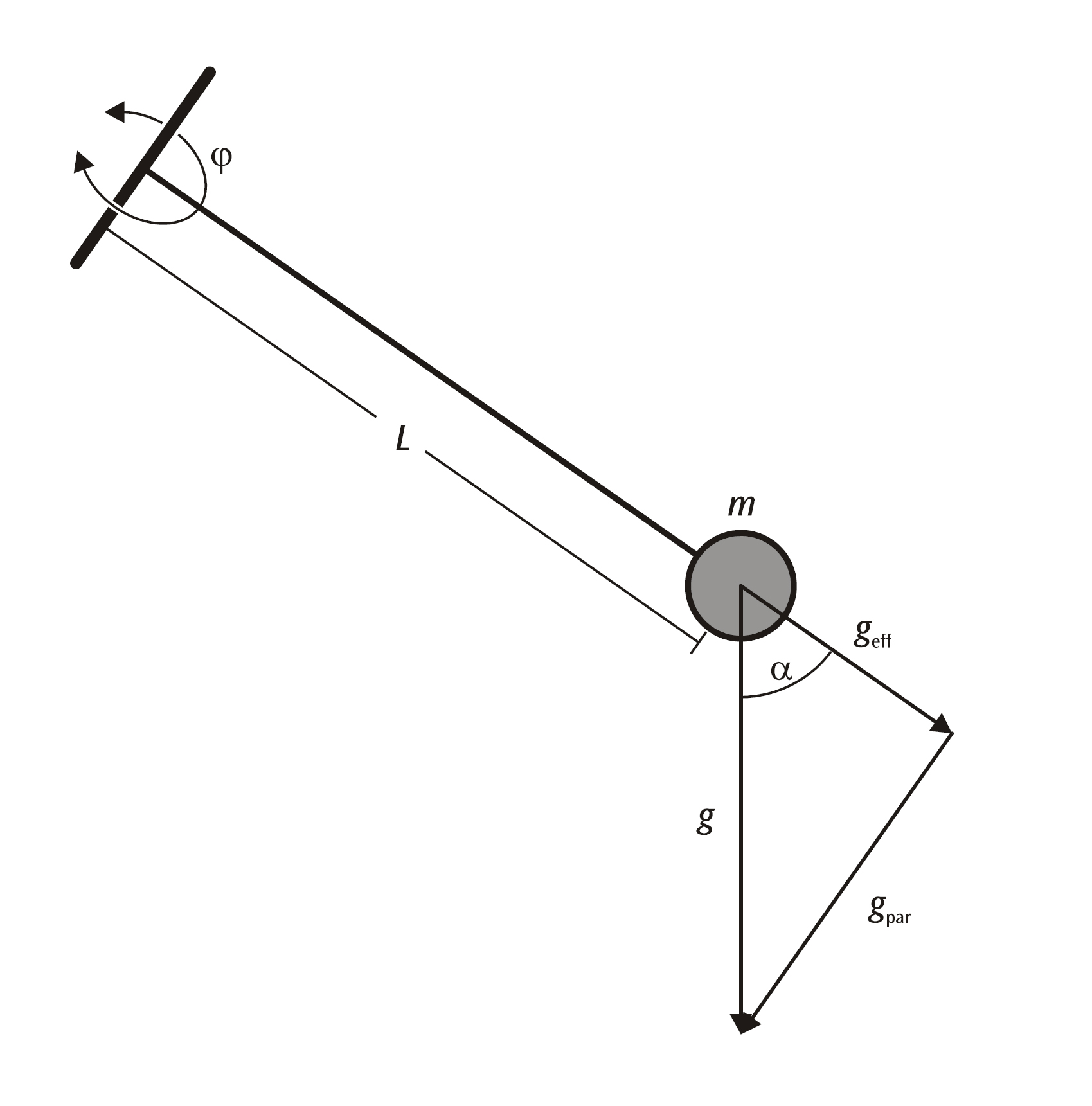Assumption in Hanoi g = 9.81.

With α = 0. Calculation of the effective pendulum length Leff for L = 300mm and L = 445mm.

geff  = g = 9.81

Use formula (2):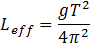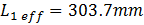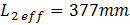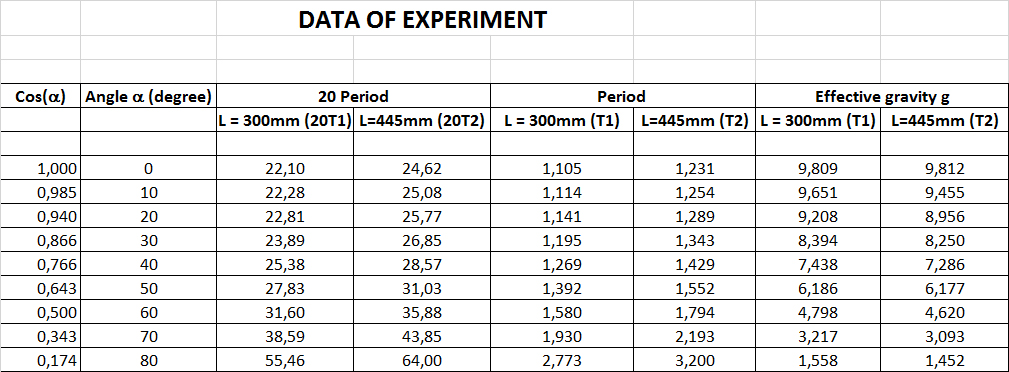Plots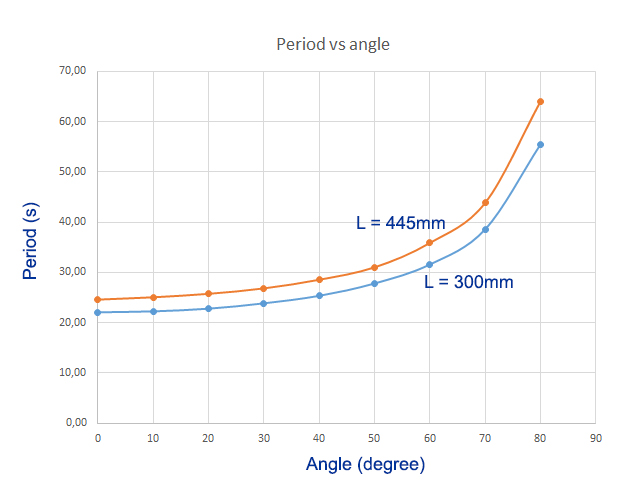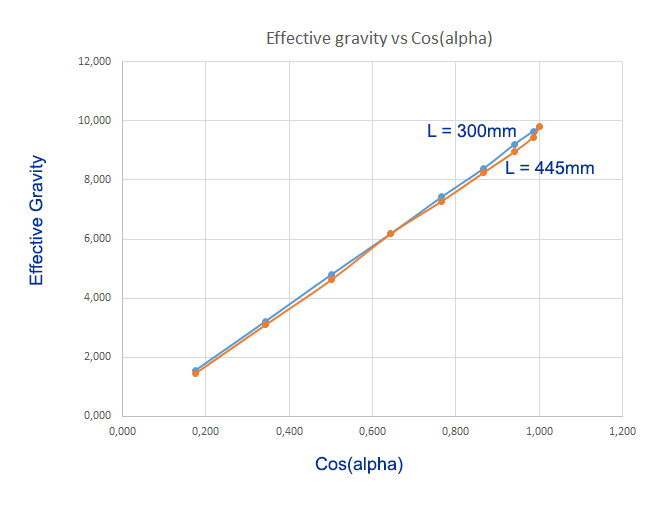gmoon = 16.6% x g = 1.625

Based on tables and graphs
We calculate it with a rotation angle of 79 degrees
The pendulum will swing like it is on the moon